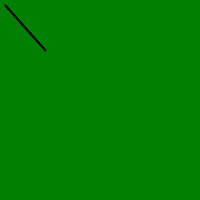# Wand Drawing() function in Python

• Last Updated : 11 May, 2020

Another module for wand is wand.drawing. This module provides us with some very basic drawing functions. wand.drawing.Drawing object buffers instructions for drawing shapes into images, and then it can draw these shapes into zero or more images.

Syntax : with Drawing() as draw:

Attention geek! Strengthen your foundations with the Python Programming Foundation Course and learn the basics.

To begin with, your interview preparations Enhance your Data Structures concepts with the Python DS Course. And to begin with your Machine Learning Journey, join the Machine Learning - Basic Level Course

Note: In the above syntax “as draw” is just a nomenclature and can be any string as needed. The Drawing function in Python wand take has no parameter.

Example #1:

 `# Import important objects from wand library``from` `wand.image ``import` `Image``from` `wand.drawing ``import` `Drawing``from` `wand.color ``import` `Color`` ` `# Create draw object using Drawing() function``with Drawing() as draw:``    ``with Image(width ``=` `200``,``               ``height ``=` `200``,``               ``background ``=` `Color(``'green'``)) as img:``        ``draw(img)``        ``img.save(filename ``=``'empty.gif'``)`

Output Image:Let’s draw a line

Example #2:

 `# Import important objects from wand library``from` `wand.image ``import` `Image``from` `wand.drawing ``import` `Drawing``from` `wand.color ``import` `Color`` ` `# Create draw object using Drawing() function``with Drawing() as draw:``    ``draw.stroke_color ``=` `Color(``'black'``)``    ``draw.stroke_width ``=` `2``    ``draw.line((``5``, ``5``), (``45``, ``50``))``    ``with Image(width ``=` `200``,``               ``height ``=` `200``,``               ``background ``=` `Color(``'green'``)) as img:``        ``draw.draw(img)``        ``img.save(filename ``=``'line.gif'``)`

Output Image:My Personal Notes arrow_drop_up